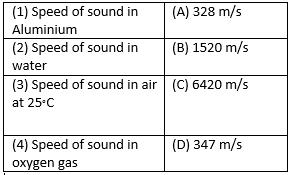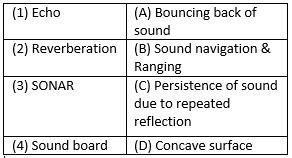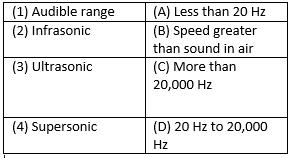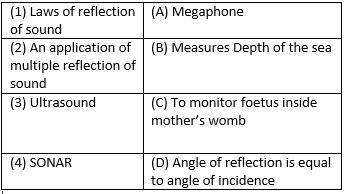Courses

# Test: Sound - 4

## 25 Questions MCQ Test Science Class 9 | Test: Sound - 4

Description
This mock test of Test: Sound - 4 for UPSC helps you for every UPSC entrance exam. This contains 25 Multiple Choice Questions for UPSC Test: Sound - 4 (mcq) to study with solutions a complete question bank. The solved questions answers in this Test: Sound - 4 quiz give you a good mix of easy questions and tough questions. UPSC students definitely take this Test: Sound - 4 exercise for a better result in the exam. You can find other Test: Sound - 4 extra questions, long questions & short questions for UPSC on EduRev as well by searching above.
QUESTION: 1

### Crests and troughs are formed in

Solution:

Crests and troughs are formed in transverse wave. The upward movement from mean position is called crest and downward movement is called troughs.

QUESTION: 2

### Statement A: Speed of sound is same at different temperature  Statement B: Loudness is measured in decibels.  Which of the two statements is true?

Solution:

Speed of sound is different at different temperature. with increase in temperature, speed of sound increases. Loudness of sound is measured in decibels.

QUESTION: 3

### Match the following with correct response.Solution:

Speed of sound in aluminum = 6420 m /s
Speed of sound in water = 1520 m /s
Speed of sound in air at 25° C = 347 m/s
Speed of sound in oxygen gas = 328 m/s

QUESTION: 4

Principle of reflection of sound is used in

Solution:

The principle of reflection of sound is used in megaphone, sound boards and stethoscope and other instruments.

QUESTION: 5

X – rays, light rays are electromagnetic waves is a

Solution:

X-rays, light rays are electrostatic waves. These waves do not require material medium for its propagation.

QUESTION: 6

Frequency of infrasonic waves is ____________ than frequency of ultrasound waves.

Solution:

Frequency of infrasonic waves is less than frequency of ultrasound waves. Frequency of infrasonic waves are less than 20Hz and frequency of ultrasonic waves are greater than 20,000Hz.

QUESTION: 7

“Due to the high power of ultrasound waves. It is used for breaking and cutting objects” The above statement is

Solution:

Ultrasound waves vibrate at frequency higher than 20,000Hz. Vibration at such a high speed can be used to breaking and cutting objects like stone in kidney.

QUESTION: 8

Match the following with correct response.Solution:

The correct option is B.

Echo -  the repetition of a sound caused by reflection of sound waves.

A reverberation, or reverb, is created when a sound or signal is reflected causing numerous reflections to build up and then decay as the sound is absorbed by the surfaces of objects in the space – which could include furniture, people, and air.

Sonar is a technique that uses sound propagation to navigate, communicate with or detect objects on or under the surface of the water, such as other vessels.

The concave surface of the sound board reflects the sound waves of the speaker towards the audience and hence prevents the spreading of sound in various directions. Due to this, sound is distributed uniformly throughout the hall and even the persons sitting at the back of the hall can hear the speech easily.

QUESTION: 9

Sound wave is a

Solution:

Sound wave propagates as compressions & rarefactions in the medium. Sound waves are longitudinal waves.

QUESTION: 10

The upper limit of frequency of sound waves audible to human beings are

Solution:

The range of hearing of normal human being is form 20 Hz to 20,000Hz. This range is called as audible range of human beings.

QUESTION: 11

Statement A: Human beings are ultrasound deaf
Statement B: Ultrasonic waves can travel easily in solid, liquid and gases.
Which of the two statements is true?

Solution:

Human beings are ultrasound deaf as human are not able to hear frequency of sound above 20,000Hz. Ultrasonic waves can travel in solid, liquid and gas but reflect backs in presence of obstruction.

QUESTION: 12

Match the following with correct response.Solution:
QUESTION: 13

The maximum displacement of the particle of the mean from its mean position is called the

Solution:

The maximum displacement from the particle of the mean position is called the Amplitude. Loudness of sound depends upon amplitude.

QUESTION: 14

The distance between a consecutive crest and trough is x. The wavelength of the wave is

Solution:

The wavelength is the distance between two consecutive crest or trough in case of transverse wave. Here, distance between consecutive crest and trough is x, so, wavelength will be 2x.

QUESTION: 15

Match the following with correct response.Solution:
QUESTION: 16

An echo is heard when-
A. Minimum distance is 17m
B. Minimum time gap between two sounds is 1/10th of second
C. Minimum distance is 18m
D. Minimum time gap between two sounds is 1/100th of second

Solution:

Two conditions for echo to be heard are as follows:-

1. 17 m minimum distance should be there.
2. Persistence of hearing is 0.1 secor minimum time gap between two sounds is 1/10 second.

QUESTION: 17

Which wave property determines loudness?

Solution:

Loudness of sound depends upon amplitude. Amplitude is the maximum displacement from mean position.

QUESTION: 18

Children can hear sound of frequency up to

Solution:

Children can hear sound of frequency up to 25,000Hz which is more than normal adult hearing range.

QUESTION: 19

Ultra sound is produced by
A. Tortoise
B. Bats
C. Dolphins
D. Dogs

Solution:

The correct option is D.
Animals such as bats,tortoises, dogs  and dolphins send out ultrasound waves and use their echoes, or reflected waves, to identify the locations of objects they cannot see. This is called echolocation. Animals use echolocation to find prey and avoid running into objects in the dark.

QUESTION: 20

The eardrum is a

Solution:

The eardrum is a stretched membranous structure at the junction of outer ear and middle ear. Vibration of eardrum causes production of sound that we hear.

QUESTION: 21

A source produces 20 crests and 20 troughs in 0.2 second. Find the frequency of the wave.

Solution:

Time–period = 0.2/20 = 0.01 second. Frequency = 1/time–period = 1/0.01 = 100Hz.

QUESTION: 22

A wave moves a distance of 10m in 0.05 second. Find the wavelength of the wave if frequency is 200Hz?

Solution:

Speed = distance/time, 10m/0.05s = 200m/s. wavelength = wave speed / frequency = 200 ms−1/200Hz = 1m.

QUESTION: 23

A bat hears the echo of its squeak after 0.1 second. How far is the obstacle from the bat? Speed of sound is 344 m/s.

Solution:

Speed of sound = 344m/s. time taken to hear echo = 0.1s. 2×distance0.1s. 2×distance = speed×time. 2×d =344×0.1 = 34.4 m. d = 17.2m.

QUESTION: 24

Cochlea, semicircular canals and the auditory nerve are associated with

Solution:

Cochlea, semicircular canals and auditory nerve are associated with inner ear.

QUESTION: 25

“Wave motion shifts mass from one region to another” The above statement is

Solution:

Wave motion shifts wave energy from one region to another region. Mass is not transferred during wave motion.